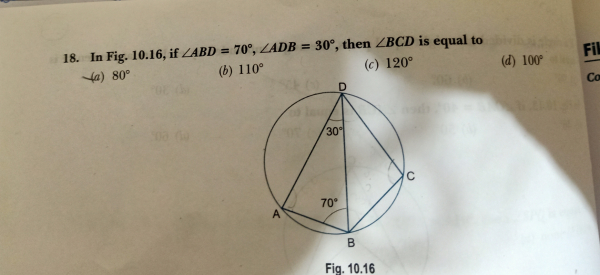"
">

# Find the measure of $\angle BCD$."

Given:

$\angle ABD=70^o, \angle ADB=30^o$.

To do:

We have to find $\angle BCD$.

Solution:

In $\vartriangle ADB$,

$\angle ABD+\angle ADB+\angle BAD=180^o$

$70^o+30^o+\angle BAD=180^o$

$\angle BAD=(180-100)^o=80^o$

We know that,

The sum of angles in opposite segments of a circle is $180^o$.

Therefore,

$\angle BAD+\angle BCD=180^o$

$80^o+\angle BCD=180^o$

$\angle BCD=(180-80)^o$

$\angle BCD=100^o$

The measure of $\angle BCD$ is $100^o$.

Updated on: 10-Oct-2022

242 Views